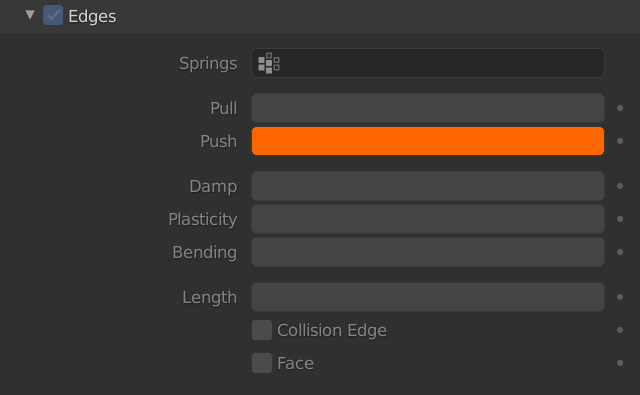# Push

– This parameter let you set compressibility of the edges.
– In a Soft Body each edge is considered as a spring (no stretch and no squeeze in the original edge length which you designed as a part of your mesh):– A low value means very compressible edge.
– A high value means a less compressible edge.

– In this example I created five vertices connected by one edge. See how different values of Push affect the stiffness of five five edges (visualized here as springs).
– The vertices on the top are free to move (weight=0) under the gravity force.
– The vertices on the bottom are fixed (weight=1)